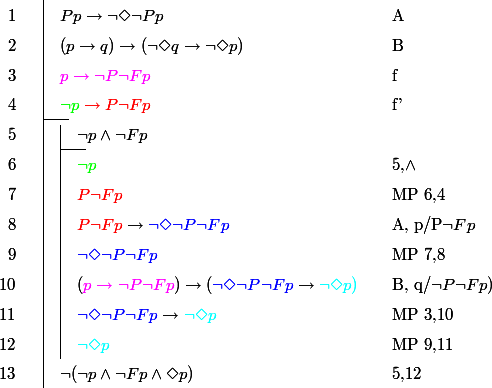# Mathtex

Summary:Add markup to display and embed several lines of mathematical formulas in wiki pages
Version:2008-10-11
Prerequisites:pmwiki 2.0 and later, latex and (dvipng or dvips+convert) installed on the server
Status:testing
Maintainer:felie
Categories: Markup Math
Discussion: Mathtex-Talk?

## Questions answered by this recipe

• Can PmWiki be used to display long mathematical sequences, logical demonstrations (in Fitch mode)?

This section is optional; use it to indicate the types of questions (if any) this recipe is intended to answer.

## Description

MathTex replace MimeTex for those who have latex installed on ther server. MathTex recipe permits several lines logical or mathematical demonstrations.

## Installation

The homesite of MathTex is: [(approve links) edit diff]

GPL licenced written in C.

As MimeTex, MathTex run in cgi

for a simple usage, you can replace occurrences of mimtex by mathtex, after installation of the MathTex script in the cgi-bin directory.

Edit the c code to set dvipng or dvips+convert locations.

Compile the cgi by

  cc mathtex.c -o mathtex.cgi


You can add \usepackage{yourpackage} directly in the code after line 1023 and recompile the cgi

### Simply replace MimeTex for simple usage

Put the mathtex.cgi in the cgi-bin directory of your server

For those who have LaTex installed on their server!

  mv mimetex.cgi mimetex.old
ln -s mathtex.cgi mimetex.cgi


(a symbolic link is set to mathtex.cgi, calling mimetex is calling mathtex)

### Customisation to manage serveral line LaTeX scripts

I prepare a MathTex script from Pm MimeTex script.

The idea of this script is simply to replace the Markup {$latex$} by {$[= latex =]$} to manage several lines.

place the mathtex.php script into the cookbook directory

in the local/config.php, add the line

  include_once('cookbook/mathtex.php');


set the values to the variables in the beginnine of the script (to run the cgi and to find the cache)

## Sample

the following code: the code use the fitch and xcolor package

{$[= \begin{fitch} \fa Pp \to \neg\Diamond\neg{Pp} & A \\ \fa (p \to q) \to (\neg\Diamond q \to \neg\Diamond p) & B \\ \fa \color{magenta} p \to \neg{P\neg{Fp}} & f \\ \fj \color{green} \neg{p} \color{red} \to P\neg{F{p}} & f' \\ \fa\fh \neg{p} \land \neg{Fp} & \\ \fa\fa \color{green} \neg{p} & 5,\land\\ \fa\fa \color{red} P\neg{Fp} & MP 6,4\\ \fa\fa \color{red} P\neg{Fp} \color{black} \to \color{blue} \neg\Diamond\neg{P\neg{Fp}} & A, p/P\neg{Fp} \\ \fa\fa \color{blue} \neg\Diamond\neg{P\neg{Fp}} & MP 7,8 \\ \fa\fa ( \color{magenta} p \to \neg{P\neg{Fp}} \color{black} ) \to ( \color{blue} \neg\Diamond\neg{P\neg{Fp}} \color{black} \to \color{cyan} \neg\Diamond{p}) & B, q/\neg{P\neg{Fp}}) \\ \fa\fa \color{blue} \neg\Diamond\neg{P\neg{Fp}} \color{black} \to \color{cyan} \neg\Diamond{p} & MP 3,10 \\ \fa\fa \color{cyan} \neg\Diamond{p} & MP 9,11 \\ \fa \neg(\neg{p} \land \neg{Fp} \land\Diamond{p}) & 5,12 \\ \end{fitch} =]$}

give:## Release Notes

This first version is for testing. Maybe it is possible to do more simple Markup for several lines, I don't know.

If the recipe has multiple releases, then release notes can be placed here. Note that it's often easier for people to work with "release dates" instead of "version numbers".

Cookbook /
AMmathjax  Add markup to embed math using ASCII MATH by MathJax. (Stable)
ASCIIMath  Display MathML rendered ascii formula into PmWiki 2.x pages (Stable)
Formula  Lightweight rendering of mathematical formulas in wiki pages (Beta)
FractionsPlus  Adds simple markup to write fractions. (Maintained)
JsMath  Add markup to display and embed mathematical formulas in wiki pages using TeX. (Obsolete - use MathJax)
LaTeXMathJax  MathJax support for PmWiki (stable)
LaTeXMathML  Translating LaTeX math notation dynamically to Presentation MathML (Stable)
LinuxTex  Enable TeX markup
LuaExecute  Lets Lua scripts dynamically generate parts of your wiki page (Beta)
MathJax  Add markup to embed math using TeX syntax. (Stable)
MimeTeX  Add markup to display and embed mathematical formulas in wiki pages (Stable)
SageCell  This allows you to embed an interactive SageCell into your webpage. Sage is an open source alternative to MathLab, Mathematica, and Maple. (Stable)
SimpleJsMath  Notes on modifying the jsMath for a skin (not a complete recipe)
TrueLatex  Enables interpretation and rendering of real LaTeX markups in PmWiki. Support for XeLaTex included in v1.8. (Working perfectly. Very simple to use, customizable and comes with lots of options.)

felie